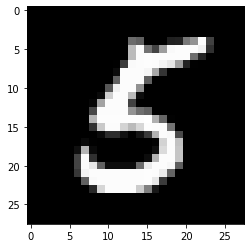# 自定义联合算法，第 2 部分：实现联合平均

## 准备工作

``````!pip install --quiet --upgrade tensorflow-federated-nightly

import nest_asyncio
nest_asyncio.apply()
``````
``````import collections

import numpy as np
import tensorflow as tf
import tensorflow_federated as tff

# TODO(b/148678573,b/148685415): must use the reference context because it
# supports unbounded references and tff.sequence_* intrinsics.
tff.backends.reference.set_reference_context()
``````
``````@tff.federated_computation
def hello_world():
return 'Hello, World!'

hello_world()
``````
```'Hello, World!'
```

## 实现联合平均

### 准备联合数据集

``````mnist_train, mnist_test = tf.keras.datasets.mnist.load_data()
``````
```Downloading data from https://storage.googleapis.com/tensorflow/tf-keras-datasets/mnist.npz
11493376/11490434 [==============================] - 0s 0us/step
11501568/11490434 [==============================] - 0s 0us/step
```
``````[(x.dtype, x.shape) for x in mnist_train]
``````
```[(dtype('uint8'), (60000, 28, 28)), (dtype('uint8'), (60000,))]
```

``````NUM_EXAMPLES_PER_USER = 1000
BATCH_SIZE = 100

def get_data_for_digit(source, digit):
output_sequence = []
all_samples = [i for i, d in enumerate(source) if d == digit]
for i in range(0, min(len(all_samples), NUM_EXAMPLES_PER_USER), BATCH_SIZE):
batch_samples = all_samples[i:i + BATCH_SIZE]
output_sequence.append({
'x':
np.array([source[i].flatten() / 255.0 for i in batch_samples],
dtype=np.float32),
'y':
np.array([source[i] for i in batch_samples], dtype=np.int32)
})
return output_sequence

federated_train_data = [get_data_for_digit(mnist_train, d) for d in range(10)]

federated_test_data = [get_data_for_digit(mnist_test, d) for d in range(10)]
``````

``````federated_train_data[-1]['y']
``````
```array([5, 5, 5, 5, 5, 5, 5, 5, 5, 5, 5, 5, 5, 5, 5, 5, 5, 5, 5, 5, 5, 5,
5, 5, 5, 5, 5, 5, 5, 5, 5, 5, 5, 5, 5, 5, 5, 5, 5, 5, 5, 5, 5, 5,
5, 5, 5, 5, 5, 5, 5, 5, 5, 5, 5, 5, 5, 5, 5, 5, 5, 5, 5, 5, 5, 5,
5, 5, 5, 5, 5, 5, 5, 5, 5, 5, 5, 5, 5, 5, 5, 5, 5, 5, 5, 5, 5, 5,
5, 5, 5, 5, 5, 5, 5, 5, 5, 5, 5, 5], dtype=int32)
```

``````from matplotlib import pyplot as plt

plt.imshow(federated_train_data[-1]['x'][-1].reshape(28, 28), cmap='gray')
plt.grid(False)
plt.show()
``````### 定义损失函数

``````BATCH_SPEC = collections.OrderedDict(
x=tf.TensorSpec(shape=[None, 784], dtype=tf.float32),
y=tf.TensorSpec(shape=[None], dtype=tf.int32))
BATCH_TYPE = tff.to_type(BATCH_SPEC)

str(BATCH_TYPE)
``````
```'<x=float32[?,784],y=int32[?]>'
```

``````MODEL_SPEC = collections.OrderedDict(
weights=tf.TensorSpec(shape=[784, 10], dtype=tf.float32),
bias=tf.TensorSpec(shape=, dtype=tf.float32))
MODEL_TYPE = tff.to_type(MODEL_SPEC)

print(MODEL_TYPE)
``````
```<weights=float32[784,10],bias=float32>
```

``````# NOTE: `forward_pass` is defined separately from `batch_loss` so that it can
# be later called from within another tf.function. Necessary because a
# @tf.function  decorated method cannot invoke a @tff.tf_computation.

@tf.function
def forward_pass(model, batch):
predicted_y = tf.nn.softmax(
tf.matmul(batch['x'], model['weights']) + model['bias'])
return -tf.reduce_mean(
tf.reduce_sum(
tf.one_hot(batch['y'], 10) * tf.math.log(predicted_y), axis=))

@tff.tf_computation(MODEL_TYPE, BATCH_TYPE)
def batch_loss(model, batch):
return forward_pass(model, batch)
``````

``````str(batch_loss.type_signature)
``````
```'(<<weights=float32[784,10],bias=float32>,<x=float32[?,784],y=int32[?]>> -> float32)'
```

``````initial_model = collections.OrderedDict(
weights=np.zeros([784, 10], dtype=np.float32),
bias=np.zeros(, dtype=np.float32))

sample_batch = federated_train_data[-1]

batch_loss(initial_model, sample_batch)
``````
```2.3025854
```

### 单个批次上的梯度下降

``````@tff.tf_computation(MODEL_TYPE, BATCH_TYPE, tf.float32)
def batch_train(initial_model, batch, learning_rate):
# Define a group of model variables and set them to `initial_model`. Must
# be defined outside the @tf.function.
model_vars = collections.OrderedDict([
(name, tf.Variable(name=name, initial_value=value))
for name, value in initial_model.items()
])
optimizer = tf.keras.optimizers.SGD(learning_rate)

@tf.function
def _train_on_batch(model_vars, batch):
# Perform one step of gradient descent using loss from `batch_loss`.
loss = forward_pass(model_vars, batch)
return model_vars

return _train_on_batch(model_vars, batch)
``````
``````str(batch_train.type_signature)
``````
```'(<<weights=float32[784,10],bias=float32>,<x=float32[?,784],y=int32[?]>,float32> -> <weights=float32[784,10],bias=float32>)'
```

``````model = initial_model
losses = []
for _ in range(5):
model = batch_train(model, sample_batch, 0.1)
losses.append(batch_loss(model, sample_batch))
``````
``````losses
``````
```[0.19690022, 0.13176313, 0.10113226, 0.082738124, 0.0703014]
```

### 本地数据序列上的梯度下降

``````LOCAL_DATA_TYPE = tff.SequenceType(BATCH_TYPE)

@tff.federated_computation(MODEL_TYPE, tf.float32, LOCAL_DATA_TYPE)
def local_train(initial_model, learning_rate, all_batches):

# Mapping function to apply to each batch.
@tff.federated_computation(MODEL_TYPE, BATCH_TYPE)
def batch_fn(model, batch):
return batch_train(model, batch, learning_rate)

return tff.sequence_reduce(all_batches, initial_model, batch_fn)
``````
``````str(local_train.type_signature)
``````
```'(<<weights=float32[784,10],bias=float32>,float32,<x=float32[?,784],y=int32[?]>*> -> <weights=float32[784,10],bias=float32>)'
```

``````locally_trained_model = local_train(initial_model, 0.1, federated_train_data)
``````

### 本地评估

``````@tff.federated_computation(MODEL_TYPE, LOCAL_DATA_TYPE)
def local_eval(model, all_batches):
# TODO(b/120157713): Replace with `tff.sequence_average()` once implemented.
return tff.sequence_sum(
tff.sequence_map(
tff.federated_computation(lambda b: batch_loss(model, b), BATCH_TYPE),
all_batches))
``````
``````str(local_eval.type_signature)
``````
```'(<<weights=float32[784,10],bias=float32>,<x=float32[?,784],y=int32[?]>*> -> float32)'
```

``````print('initial_model loss =', local_eval(initial_model,
federated_train_data))
print('locally_trained_model loss =',
local_eval(locally_trained_model, federated_train_data))
``````
```initial_model loss = 23.025854
locally_trained_model loss = 0.4348469
```

``````print('initial_model loss =', local_eval(initial_model,
federated_train_data))
print('locally_trained_model loss =',
local_eval(locally_trained_model, federated_train_data))
``````
```initial_model loss = 23.025854
locally_trained_model loss = 74.50075
```

### 联合评估

``````SERVER_MODEL_TYPE = tff.type_at_server(MODEL_TYPE)
CLIENT_DATA_TYPE = tff.type_at_clients(LOCAL_DATA_TYPE)
``````

``````@tff.federated_computation(SERVER_MODEL_TYPE, CLIENT_DATA_TYPE)
def federated_eval(model, data):
return tff.federated_mean(
``````

``````print('initial_model loss =', federated_eval(initial_model,
federated_train_data))
print('locally_trained_model loss =',
federated_eval(locally_trained_model, federated_train_data))
``````
```initial_model loss = 23.025852
locally_trained_model loss = 54.432625
```

### 联合训练

``````SERVER_FLOAT_TYPE = tff.type_at_server(tf.float32)

@tff.federated_computation(SERVER_MODEL_TYPE, SERVER_FLOAT_TYPE,
CLIENT_DATA_TYPE)
def federated_train(model, learning_rate, data):
return tff.federated_mean(
tff.federated_map(local_train, [
]))
``````

``````model = initial_model
learning_rate = 0.1
for round_num in range(5):
model = federated_train(model, learning_rate, federated_train_data)
learning_rate = learning_rate * 0.9
loss = federated_eval(model, federated_train_data)
print('round {}, loss={}'.format(round_num, loss))
``````
```round 0, loss=21.60552406311035
round 1, loss=20.365678787231445
round 2, loss=19.27480125427246
round 3, loss=18.31110954284668
round 4, loss=17.45725440979004
```

``````print('initial_model test loss =',
federated_eval(initial_model, federated_test_data))
print('trained_model test loss =', federated_eval(model, federated_test_data))
``````
```initial_model test loss = 22.795593
trained_model test loss = 17.278767
```

[{ "type": "thumb-down", "id": "missingTheInformationINeed", "label":"没有我需要的信息" },{ "type": "thumb-down", "id": "tooComplicatedTooManySteps", "label":"太复杂/步骤太多" },{ "type": "thumb-down", "id": "outOfDate", "label":"内容需要更新" },{ "type": "thumb-down", "id": "translationIssue", "label":"翻译问题" },{ "type": "thumb-down", "id": "samplesCodeIssue", "label":"示例/代码问题" },{ "type": "thumb-down", "id": "otherDown", "label":"其他" }]
[{ "type": "thumb-up", "id": "easyToUnderstand", "label":"易于理解" },{ "type": "thumb-up", "id": "solvedMyProblem", "label":"解决了我的问题" },{ "type": "thumb-up", "id": "otherUp", "label":"其他" }]